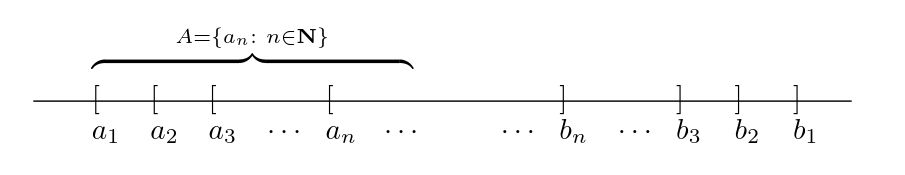Show Question
Math and science::Analysis::Tao::05. The real numbers

# Nested intervals property for reals

### Nested interval property

A sequence of nested closed intervals of reals has a nonempty intersection.

In other words, if one considers a sequence of nested closed intervals $$(I_n)_{n=0}^{\infty}$$ such that for each $$n \in \mathbb{N}$$, $$I_n = [a_n, b_n]$$ for some $$a_n, b_n \in \mathbb{R}$$ and $$I_{n+1} \subseteq I_n$$, then It holds that $$\cap_{n=1}^{\infty} I_n \neq \emptyset$$.

Proof on the reverse.

### Proof

The proof uses the property that sets of reals have a supremum; this is used to find an $$x \in \mathbb{R}$$ such that $$x \in \cap_{n=0}^{\infty} I_n$$.

Proof. Let $$A = \{a_n : n \in \mathbb{N} \}$$ be the set of left-endpoints for the intervals. Note that for every $$i \in \mathbb{N}$$, $$b_i$$ is an upper bound for $$A$$. Let $$x = \sup A$$.

• $$\forall i \in \mathbb{N}, x \le b_i$$, as $$x$$ is the least upper bound for $$A$$, and every $$b_i$$ is an upper bound.
• $$\forall i \in \mathbb{N}, x \ge a_i$$, as $$x$$ is an upper bound for $$A$$ and all $$a_i \in A$$.

So, we have $$\forall i \in \mathbb{N}, a_n \le x \le b_n$$, which means that $$\forall i \in \mathbb{N}, x \in I_n$$, and furthermore, $$x \in \cap_{n=0}^{\infty} I_n$$ meaning that this intersection is not empty.

#### Visualization of the interval endpointsAbbott, p20